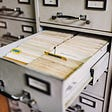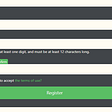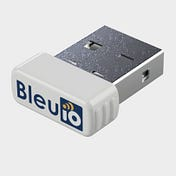# C# Desktop application to get real-time BLE data from Air Quality Sensor

`using System;using System.IO.Ports;using System.Linq;using System.Text;using System.Windows.Forms;using System.Collections;using System.Threading;namespace WindowsFormsApp1{        public partial class Form1 : Form    {        //Connect to Serial port        //Replace port number to your comport        SerialPort mySerialPort = new SerialPort("COM18", 57600, Parity.None, 8, StopBits.One);        ArrayList device = new ArrayList();        string ScannedData = "";        public string selectedDevices = "";        string advData = "";        // HibouAir Advertise data decrypt and show output        public String ParseSensorData(string input)        {            int counter = 17;            string theData = " SensorID : " + input[counter + 1] +              input[counter + 2] +              input[counter + 3] +              input[counter + 4] +              input[counter + 5] +              input[counter + 6] + "\n\n " +              "Pressure : " + Convert.ToInt32(input[counter + 13].ToString() + input[counter + 14].ToString() + input[counter + 11].ToString() + input[counter + 12].ToString(), 16) / 10 + " mbar\n\n " +              "Temperature : " + Convert.ToInt32(input[counter + 17].ToString() + input[counter + 18].ToString() + input[counter + 15].ToString() + input[counter + 16].ToString(), 16) / 10 + " °C\n\n " +              "Humidity : " + Convert.ToInt32(input[counter + 21].ToString() + input[counter + 22].ToString() + input[counter + 19].ToString() + input[counter + 20].ToString(), 16) / 10 + " %rH\n\n " +              "VOC : " + Convert.ToInt32(input[counter + 25].ToString() + input[counter + 26].ToString() + input[counter + 23].ToString() + input[counter + 24].ToString(), 16) / 10 + "\n\n " +              "ALS : " + Convert.ToInt32(input[counter + 9].ToString() + input[counter + 10].ToString() + input[counter + 7].ToString() + input[counter + 8].ToString(), 16) + " Lux\n\n " +              "PM 1.0 : " + Convert.ToInt32(input[counter + 29].ToString() + input[counter + 30].ToString() + input[counter + 27].ToString() + input[counter + 28].ToString(), 16) / 10 + " µg/m³\n\n " +              "PM 2.5 : " + Convert.ToInt32(input[counter + 33].ToString() + input[counter + 34].ToString() + input[counter + 31].ToString() + input[counter + 32].ToString(), 16) / 10 + " µg/m³\n\n " +              "PM 10 : " + Convert.ToInt32(input[counter + 37].ToString() + input[counter + 38].ToString() + input[counter + 35].ToString() + input[counter + 36].ToString(), 16) / 10 + " µg/m³"              ;            sensor_op.Text = theData.ToString();            return theData;        }        public Form1()        {            InitializeComponent();                mySerialPort.DataReceived += new SerialDataReceivedEventHandler(mySerialPort_DataReceived);            mySerialPort.Open();            ArrayList device = new ArrayList();        }                //Store response from the dongle        private void mySerialPort_DataReceived(object sender, SerialDataReceivedEventArgs e)        {                        SerialPort sp = (SerialPort)sender;            string s = sp.ReadExisting();            if (s.Contains("RSSI") && s.Contains("(HibouAIR)") )            {                device.Add(s);                            }            if (selectedDevices != "")            {                label1.Invoke(new EventHandler(delegate { label1.Text = selectedDevices.Remove(0, 3); }));                            if (s.Contains("[ADV]"))                {                    ScannedData=s;                                       // output_data.Invoke(new EventHandler(delegate { output_data.Text += "TT: " + s + "\r\n"; }));                }                        }            //output_data.Invoke(new EventHandler(delegate { output_data.Text += s + "\r\n"; }));            //lbl_output.Invoke(this.myDelegate, new Object[] { s });        }               // Disconnect from COM port        private void btn_disconnect_Click(object sender, EventArgs e)        {            mySerialPort.Close();            Environment.Exit(0);        }        private void Form1_Load(object sender, EventArgs e)        {        }        private void pictureBox1_Click(object sender, EventArgs e)        {        }        private void btn_deviceList_Click(object sender, EventArgs e)        {            var inputByte = new byte[] { 13 };            byte[] dualCmd = Encoding.UTF8.GetBytes("AT+DUAL");            dualCmd = dualCmd.Concat(inputByte).ToArray();            mySerialPort.Write(dualCmd, 0, dualCmd.Length);            byte[] gapScanCmd = Encoding.UTF8.GetBytes("AT+GAPSCAN=2");            gapScanCmd = gapScanCmd.Concat(inputByte).ToArray();            mySerialPort.Write(gapScanCmd, 0, gapScanCmd.Length);                    }        private void label1_Click(object sender, EventArgs e)        {        }        // Load devices on dropdown        private void dropdown_device_SelectedIndexChanged(object sender, EventArgs e)        {            if(dropdown_device.Text == "Load Devices")            {                                foreach (var a in device)                {                    dropdown_device.Items.Add(a);                }            }            else            {                selectedDevices = dropdown_device.Text;                string[] selectedDeviceID = selectedDevices.Split(' ');                selectedDevices = selectedDeviceID;                btnGetData.Visible = true;            }                    }        //get advertised data of selected device        private void btnGetData_Click(object sender, EventArgs e)        {            var inputByte = new byte[] { 13 };            byte[] gapScanCmd = Encoding.UTF8.GetBytes("AT+FINDSCANDATA=FF5B07");            gapScanCmd = gapScanCmd.Concat(inputByte).ToArray();            mySerialPort.Write(gapScanCmd, 0, gapScanCmd.Length);            Thread.Sleep(500);            byte[] bytes = Encoding.UTF8.GetBytes("\u0003");            bytes = bytes.Concat(inputByte).ToArray();            mySerialPort.Write(bytes, 0, bytes.Length);            var array = ScannedData.Split('\n');            int i = 0;            //filter selected device data            foreach (var a in array)            {                string kk = (string)a;                if (kk.Contains(selectedDevices.Remove(0, 3)))                {                    var bleData = array[i].Split(' ');                    advData = bleData;                    var toPrint = ParseSensorData(advData);                    break;                }                i++;            }                    }    }    }`

--

--

--

## More from bleuio

The BleuIO is Bluetooth low energy solution that can be used to create new BLE 5.0 applications in the fastest and easiest way.

Love podcasts or audiobooks? Learn on the go with our new app.

## Different Fun Commands In Linux## How to Build a Fast 404 Error Page in Kartra to Map Your Domain Name## Java to C# — C# to Java## Flutter Widget Guide — Index## Intro to Microservices## 🐊How to subscribe Fungle🐊## Jetifier Reverse Mode## bleuio

The BleuIO is Bluetooth low energy solution that can be used to create new BLE 5.0 applications in the fastest and easiest way.

## Publish a .NET Console App to Chocolatey using GitHub Actions## Top .NET development companies to outsource## .NET Code coverage on GitLab## Plotting real-time graph from Bluetooth device using C#